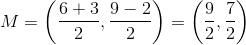# SSAT Upper Level Math : How to find the midpoint of a line segment

## Example Questions

### Example Question #1 : How To Find The Midpoint Of A Line Segment

A line segment on the coordinate plane has endpoints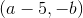and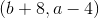. In terms ofand, as applicable, give the-coordinate of its midpoint.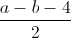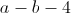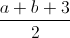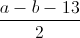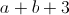Explanation:

The-coordinate of the midpoint of a line segment is the mean of the-coordinates of its endpoints. Therefore, the-coordinate is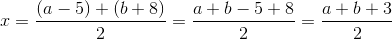.

### Example Question #2 : Midpoint Formula

A line segment on the coordinate plane has endpointsand. In terms ofand, as applicable, give the-coordinate of its midpoint.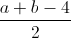Explanation:

The-coordinate of the midpoint of a line segment is the mean of the-coordinates of its endpoints. Therefore, the-coordinate is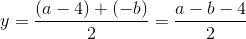.

### Example Question #3 : Midpoint Formula

A line segment has the endpoints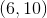and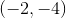. What is the midpoint of the line?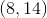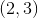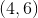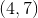Explanation:

To find the midpoint of a line, take the averages of the x-coordinates and the average of the y-coordinates.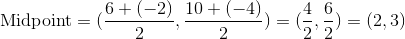### Example Question #4 : Midpoint Formula

What is the midpoint of a line segment with endpoints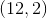and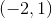?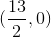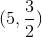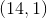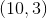Explanation:

To find the midpoint of a line, just take the average of the-coordinates and the average of the-coordinates.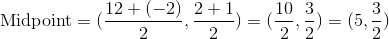### Example Question #5 : Midpoint Formula

What is the midpoint of a line segments with endpoints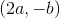and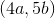?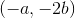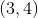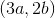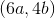Explanation:

To find the midpoint of a line segment, take the average of the-coordinates, then take the average of the-coordinates.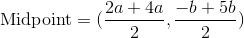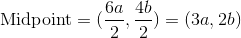### Example Question #6 : Midpoint Formula

A line has the endpoints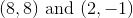. What is its midpoint?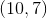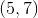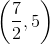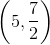Explanation:

The coordinates of the midpoint of a line are just the averages of the coordinates of the endpoints.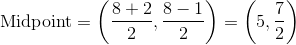### Example Question #7 : Midpoint Formula

A line segment has endpoints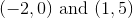. What is the midpoint of this line segment?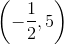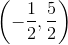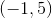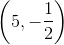Explanation:

To find the coordinates of the midpoint of a line, take the averages of the x and y coordinates of the endpoints.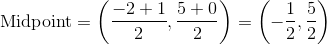### Example Question #8 : Midpoint Formula

What is the midpoint of a line if the endpoints of the line are: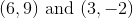?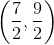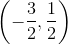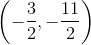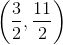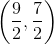Explanation:

Write the midpoint formula.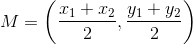Substitute the values.